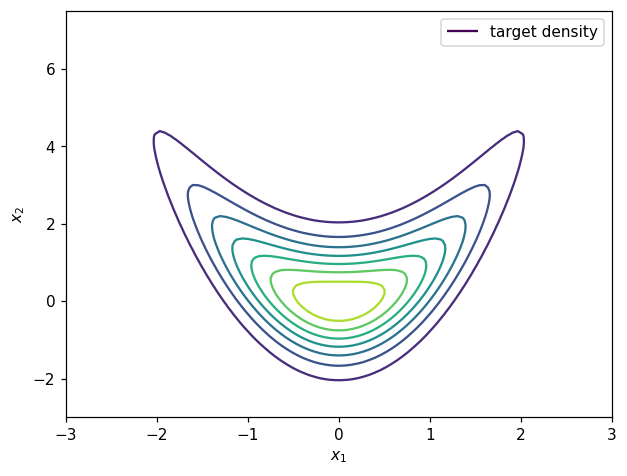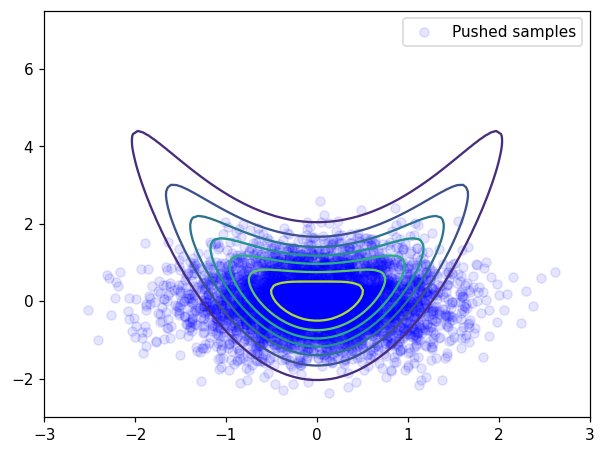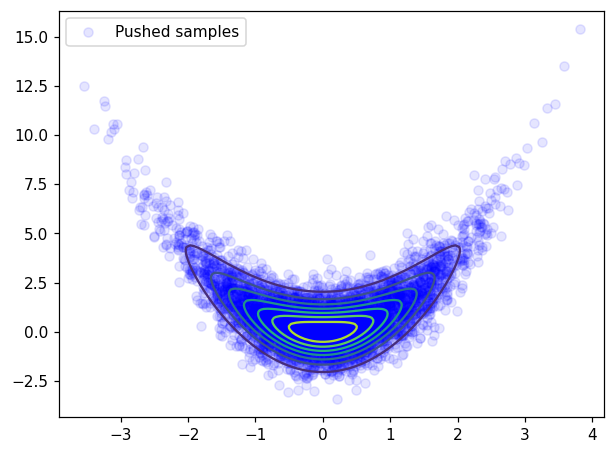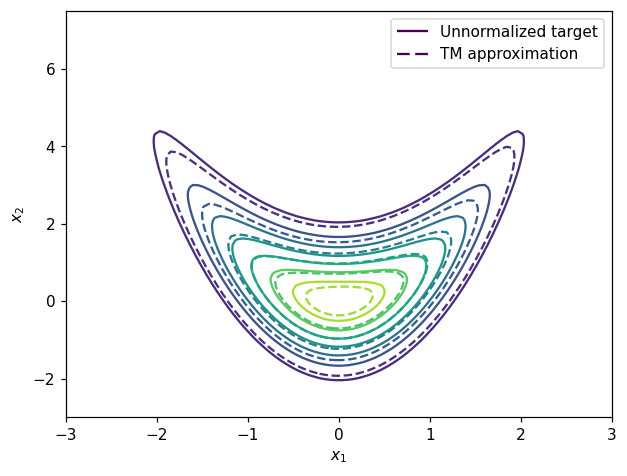Launch this notebook on on mybinder.org:# Transport Map from density#

The objective of this example is to show how a transport map can be build in MParT when the the unnormalized probability density function of the target density is known.

## Problem description#

We consider $$T(\mathbf{z};\mathbf{w})$$ a monotone triangular transport map parameterized by $$\mathbf{w}$$ (e.g., polynomial coefficients). This map which is invertible and has an invertible Jacobian for any parameter $$\mathbf{w}$$, transports samples $$\mathbf{z}^i$$ from the reference density $$\eta$$ to samples $$T(\mathbf{z}^i;\mathbf{w})$$ from the map induced density $$\tilde{\pi}_\mathbf{w}(\mathbf{z})$$ defined as:

$\tilde{\pi}_\mathbf{w}(\mathbf{z}) = \eta(T^{-1}(\mathbf{z};\mathbf{w}))|\text{det } T^{-1}(\mathbf{z};\mathbf{w})|,$

where $$\text{det } T^{-1}$$ is the determinant of the inverse map Jacobian at the point $$\mathbf{z}$$. We refer to $$\tilde{\pi}_{\mathbf{w}}(\mathbf{x})$$ as the map-induced density or pushforward distribution and will commonly interchange notation for densities and measures to use the notation $$\tilde{\pi} = T_{\sharp} \eta$$.

The objective of this example is, knowing some unnormalized target density $$\bar{\pi}$$, find the map $$T$$ that transport samples drawn from $$\eta$$ to samples drawn from the target $$\pi$$.

## Imports#

First, import MParT and other packages used in this notebook. Note that it is possible to specify the number of threads used by MParT by setting the KOKKOS_NUM_THREADS environment variable before importing MParT.

:

import numpy as np
from scipy.optimize import minimize
import matplotlib.pyplot as plt
from scipy.stats import multivariate_normal

import os

import mpart as mt
plt.rcParams['figure.dpi'] = 110

Kokkos is usingKokkos::OpenMP::initialize WARNING: You are likely oversubscribing your CPU cores.

Kokkos::OpenMP::initialize WARNING: You are likely oversubscribing your CPU cores.
Detected: 2 cores per node.
Detected: 1 MPI_ranks per node.


## Target density and exact map#

In this example we use a 2D target density known as the banana density where the unnormalized probability density, samples and the exact transport map are known.

The banana density is defined as:

$\pi(x_1,x_2) \propto N_1(x_1)\times N_1(x_2-x_1^2)$

where $$N_1$$ is the 1D standard normal density.

The exact transport map that transport the 2D standard normal density to $$\pi$$ is known as:

$\begin{split} {T}^\text{true}(z_1,z_2)= \begin{bmatrix} z_1\\ z_2 + z_1^2 \end{bmatrix}\end{split}$

Contours of the target density can be visualized as:

:

# Unnomalized target density required for objective
def target_logpdf(x):
rv1 = multivariate_normal(np.zeros(1),np.eye(1))
rv2 = multivariate_normal(np.zeros(1),np.eye(1))
logpdf1 = rv1.logpdf(x)
logpdf2 = rv2.logpdf(x-x**2)
logpdf = logpdf1 + logpdf2
return logpdf

# Grid for plotting
ngrid=100
x1_t = np.linspace(-3,3,ngrid)
x2_t = np.linspace(-3,7.5,ngrid)
xx1,xx2 = np.meshgrid(x1_t,x2_t)

xx = np.vstack((xx1.reshape(1,-1),xx2.reshape(1,-1)))

# Target contours
target_pdf_at_grid = np.exp(target_logpdf(xx))

fig, ax = plt.subplots()
CS1 = ax.contour(xx1, xx2, target_pdf_at_grid.reshape(ngrid,ngrid))
ax.set_xlabel(r'$x_1$')
ax.set_ylabel(r'$x_2$')
h1,_ = CS1.legend_elements()
legend1 = ax.legend([h1], ['target density'])
plt.show()## Map training#

### Defining objective function and its gradient#

Knowing the closed form of the unnormalized target density $$\bar{\pi}$$, the objective is to find a map-induced density $$\tilde{\pi}_{\mathbf{w}}(\mathbf{z})$$ that is a good approximation of the target $$\pi$$.

In order to characterize this posterior density, one method is to build a monotone triangular transport map $$T$$ such that the KL divergence $$D_{KL}(\eta || T^\sharp \pi)$$ is minmized. If $$T$$ is map parameterized by $$\mathbf{w}$$, the objective function derived from the discrete KL divergence reads:

$J(\mathbf{w}) = - \frac{1}{N}\sum_{i=1}^N \left( \log\pi\left(T(\mathbf{z}^i;\mathbf{w})\right) + \log \text{det }\nabla_\mathbf{z} T(\mathbf{z}^i;\mathbf{w})\right), \,\,\, \mathbf{z}^i \sim \mathcal{N}(\mathbf{0},\mathbf{I}_d),$

where $$T$$ is the transport map pushing forward the standard normal $$\mathcal{N}(\mathbf{0},\mathbf{I}_d)$$ to the target density $$\pi(\mathbf{z})$$. The gradient of this objective function reads

$\nabla_\mathbf{w} J(\mathbf{w}) = - \frac{1}{N}\sum_{i=1}^N \left( \nabla_\mathbf{w} T(\mathbf{z}^i;\mathbf{w}).\nabla_\mathbf{x}\log\pi\left(T(\mathbf{z}^i;\mathbf{w})\right) + \nabla_{\mathbf{w}}\log \text{det }\nabla_\mathbf{z} T(\mathbf{z}^i;\mathbf{w})\right), \,\,\, \mathbf{z}^i \sim \mathcal{N}(\mathbf{0},\mathbf{I}_d).$

The objective function and gradient can be defined using MParT as:

:

# KL divergence objective
def obj(coeffs, transport_map, x):
num_points = x.shape
transport_map.SetCoeffs(coeffs)
map_of_x = transport_map.Evaluate(x)
logpdf= target_logpdf(map_of_x)
log_det = transport_map.LogDeterminant(x)
return -np.sum(logpdf + log_det)/num_points

# Gradient of KL divergence objective
num_points = x.shape
transport_map.SetCoeffs(coeffs)
map_of_x = transport_map.Evaluate(x)


### Map parameterization#

For the parameterization of $$T$$ we use a total order multivariate expansion of hermite functions. Knowing $$T^\text{true}$$, any parameterization with total order greater than one will include the true solution of the map finding problem.

:

# Set-up first component and initialize map coefficients
map_options = mt.MapOptions()

total_order = 2

# Create dimension 2 triangular map
transport_map = mt.CreateTriangular(2,2,total_order,map_options)


### Approximation before optimization#

Coefficients of triangular map are set to 0 upon creation.

:

# Make reference samples for training
num_points = 10000
z = np.random.randn(2,num_points)

# Make reference samples for testing
test_z = np.random.randn(2,5000)

# Pushed samples
x = transport_map.Evaluate(test_z)

# Before optimization plot
plt.figure()
plt.contour(xx1, xx2, target_pdf_at_grid.reshape(ngrid,ngrid))
plt.scatter(x,x, facecolor='blue', alpha=0.1, label='Pushed samples')
plt.legend()
plt.show()At initialization, samples are “far” from being distributed according to the banana distribution.

Initial objective and coefficients:

:

# Print initial coeffs and objective
print('==================')
print('Starting coeffs')
print(transport_map.CoeffMap())
print('Initial objective value: {:.2E}'.format(obj(transport_map.CoeffMap(), transport_map, test_z)))
print('==================')

==================
Starting coeffs
[0. 0. 0. 0. 0. 0. 0. 0. 0.]
Initial objective value: 3.39E+00
==================


### Minimization#

:

print('==================')
options={'gtol': 1e-4, 'disp': True}
res = minimize(obj, transport_map.CoeffMap(), args=(transport_map, z), jac=grad_obj, method='BFGS', options=options)

# Print final coeffs and objective
print('Final coeffs:')
print(transport_map.CoeffMap())
print('Final objective value: {:.2E}'.format(obj(transport_map.CoeffMap(), transport_map, test_z)))
print('==================')

==================
Optimization terminated successfully.
Current function value: 2.844511
Iterations: 17
Function evaluations: 19
Final coeffs:
[-0.01108237  0.84833716  0.00631056  0.98331819  0.84867706  0.00667816
-0.00985025  0.02820473  2.22978852]
Final objective value: 2.83E+00
==================


### Approximation after optimization#

#### Pushed samples#

:

# Pushed samples
x = transport_map.Evaluate(test_z)

# After optimization plot
plt.figure()
plt.contour(xx1, xx2, target_pdf_at_grid.reshape(ngrid,ngrid))
plt.scatter(x,x, facecolor='blue', alpha=0.1, label='Pushed samples')
plt.legend()
plt.show()After optimization, pushed samples $$T(z^i)$$, $$z^i \sim \mathcal{N}(0,I)$$ are approximately distributed according to the target $$\pi$$

#### Variance diagnostic#

A commonly used accuracy check when facing computation maps from density is the so-called variance diagnostic defined as:

$\epsilon_\sigma = \frac{1}{2} \mathbb{V}\text{ar}_\rho \left[ \log \frac{\rho}{T^\sharp \bar{\pi}} \right]$

This diagnostic is asymptotically equivalent to the minimized KL divergence $$D_{KL}(\eta || T^\sharp \pi)$$ and should converge to zero when the computed map converge to the true map.

The variance diagnostic can be computed as follow:

:

def variance_diagnostic(tri_map,ref,target_logpdf,x):
ref_logpdf = ref.logpdf(x.T)
y = tri_map.Evaluate(x)
pullback_logpdf = target_logpdf(y) + tri_map.LogDeterminant(x)
diff = ref_logpdf - pullback_logpdf
expect = np.mean(diff)
var = 0.5*np.mean((diff-expect)**2)
return var

:

# Reference distribution
ref_distribution = multivariate_normal(np.zeros(2),np.eye(2));

# Compute variance diagnostic
var_diag = variance_diagnostic(transport_map,ref_distribution,target_logpdf,test_z)

# Print variance diagnostic
print('==================')
print('Variance diagnostic: {:.2E}'.format(var_diag))
print('==================')

==================
Variance diagnostic: 3.13E-04
==================


#### Pushforward density#

We can also plot the contour of the unnormalized density $$\bar{\pi}$$ and the pushforward approximation $$T_\sharp \eta$$:

:

# Pushforward definition
def push_forward_pdf(tri_map,ref,x):
xinv = tri_map.Inverse(x,x)
return np.exp(log_pdf)

map_approx_grid = push_forward_pdf(transport_map,ref_distribution,xx)

fig, ax = plt.subplots()
CS1 = ax.contour(xx1, xx2, target_pdf_at_grid.reshape(ngrid,ngrid))
CS2 = ax.contour(xx1, xx2, map_approx_grid.reshape(ngrid,ngrid),linestyles='--')
ax.set_xlabel(r'$x_1$')
ax.set_ylabel(r'$x_2$')
h1,_ = CS1.legend_elements()
h2,_ = CS2.legend_elements()
legend1 = ax.legend([h1, h2], ['Unnormalized target', 'TM approximation'])
plt.show()[ ]: